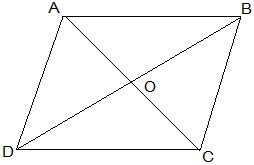Guru

# Show that the diagonals of a parallelogram divide it into four triangles of equal area. Q.3

• 0

What is the easiest way for solving the question of class 9th ncert math of exercise 9.3of math of Areas of Parallelograms and Triangles chapter of question no.3  Please help me for the best suggestion of this question Show that the diagonals of a parallelogram divide it into four triangles of equal area.

Share

1.O is the mid point of AC and BD. (diagonals of bisect each other)

In ΔABC, BO is the median.

∴ar(AOB) = ar(BOC) — (i)

also,

In ΔBCD, CO is the median.

∴ar(BOC) = ar(COD) — (ii)

In ΔACD, OD is the median.

∴ar(AOD) = ar(COD) — (iii)

In ΔABD, AO is the median.

∴ar(AOD) = ar(AOB) — (iv)

From equations (i), (ii), (iii) and (iv), we get,

ar(BOC) = ar(COD) = ar(AOD) = ar(AOB)

Hence, we get, the diagonals of a parallelogram divide it into four triangles of equal area.

• 0